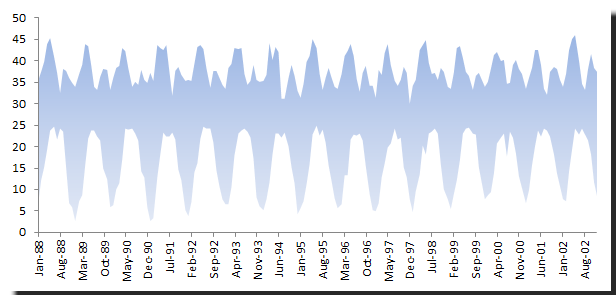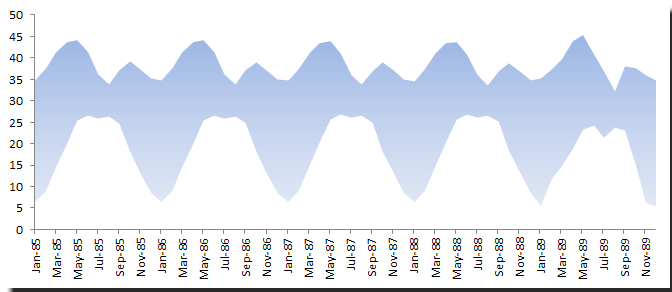# Backward Forecast

No, this is not meant as an oxymoron but rather as a question raised by one of our users. And we thought it may be interesting to others

So the story goes like this: When you have a sample time series, most of the time you would like to forecast just the future points (past the end of the sample data). But what about the ones that fall before the start of the sample? Can we predict those as well? And if so, what can we say about the forecasting process?

## Why should we care?

There are different cases where one would want to predict the past.

For example, our user had a temperature time series that was missing past observations, and he wanted the best guess for them using the dynamics detected in the same data.

For financial time series, there is no money to make here by forecasting the past (unless we have a time machine). But can’t we use the past data points and their forecasts to help us diagnose the stability of the underlying process?

In this issue, we will show how to make a backward forecast using only NumXL functions in Excel. We will also discuss the relationship between a regular time series model and an implied backward/reversed time series model.

For the data sample, we’ll use the monthly MIN‐MAX temperatures recorded in a given city from January 1988 to December 2009.

## Background

In time series, we usually express a value of a data point as a function of prior values.

$$X_t=f(X_{t-1},X_{t-2},\cdots,X_1, a_{t-1},a_{t-2},\cdots,a_1)+a_t$$

Where:

• $\{X_{t-1},X_{t-2},\cdots,X_1\}$ is a values set of past observations
• $\{a_{t-1},a_{t-2},\cdots,a_1\}$ is a set of past shocks/innovations

In order to reverse the problem we need to express the past observation values in terms of future ones.

$$X_t=g(X_{t+1},X_{t+2}\cdots X_T, a_{t+1}, a_{t+2}\cdots , a_T) + a_t$$

Where:

• $\{X_{t+1},X_{t+2}\cdots X_T\}$ are the values of future observations up to the end of the sample
• $\{a_{t+1}, a_{t+2}\cdots , a_T\}$ is a set of future shocks or innovations up to the end of the sample

When examining the two forms, you can see the backward model is basically a time series model but with the chronicle‐reversed time series $\{Y_t\}$ data, such that:

$$Y_t = X_{T-t}$$ $$X_t = Y_{T-t}$$

So, let’s reformulate the relations earlier

$$t=T-\tau$$ $$X_{T-\tau}=g(X_{T-\tau+1},X_{T-\tau+2}\cdots X_{t+\tau},a_{T-\tau+1},a_{T-\tau+2}\cdots a_{t+\tau})+a_{T-\tau}$$ $$X_{T-\tau}=g(X_{T-(\tau-1)},X_{T-(\tau-2)}\cdots X_{T-(T-t-\tau)},a_{T-(\tau-1)},a_{T-(\tau-2)}\cdots a_{T-(T-t-\tau)})+a_{T-\tau}$$ $$Y_\tau=g(Y_{\tau-1},Y_{\tau-2}\cdots Y_0,\omega_{\tau-1},\omega_{\tau-2}\cdots \omega_0)+\omega_\tau$$

where:

• $\{\omega_{\tau} \}=\{a_{T-\tau}\}$

By reversing the chronicle order of the time series we can fit a regular time series model and forecast new values as we have usually done, but we interpret them as the past values in the original time series domain.

## Application

For our sample data, we’ll use the monthly MAX‐MIN temperature (Celsius) recorded at a given city;Note that the time series exhibits 12‐month seasonality and some upward drift (trend).

The objective here is to forecast the monthly MIN‐MAX temperatures from 1984 and 1988

Note that minimal and maximum temperature time series are correlated. But for our purposes here, we’ll ignore the interdependency and forecast each time series separately.

Furthermore, we will use Winters’ triple exponential smoothing function to drive the forecast.

## Forecast

As mentioned earlier, we reversed the chronicle order of the input time series (MIN and MAX) such that the first observation is the last one and vice versa. We used the NumXL “REVERSE” function.

Next, using Holt‐Winters’ triple exponential function (TESMTH in NumXL),

1. We assumed a default value for $\{\alpha,\beta,\gamma\}$ of 0.1 and computed an in‐sample MAX forecast for each data point
2. Using the root mean squared errors (i.e. RMSE) function, we calculated the discrepancy measure between the model’s value and the sample data values
3. Using the Solver, we optimized the values of $\{\alpha,\beta,\gamma\}$ that would minimize the RMSE value. For more details about how we calibrated the coefficients, please refer to our issue on smoothing functions where we tackle the parameters’ value optimization.
4. Afterward, we used the optimal values of the TESMTH function to forecast out‐of‐sample data points.
5. Then we repeated the same procedure for the MIN time seriesIn the graph above, all observations before December 1988 were forecasted by the TESMTH function. The forecast preserved the seasonality, and the trend is very minimal.

## Conclusion

We’ve demonstrated how to do a backward forecast, which is a prediction for an observation that falls before the start of the sample data. The key step was reversing the chronicle order of the input time series before the start of the analysis.

Furthermore, the time series process of the original data is different from the process of the reversed time series.

$$x_t=\alpha +\phi x_{t-1}+ a_t$$ $$\left |\phi \right | < 1$$

To reverse the relationship,

$$x_{t-1}=\frac{-\alpha+x_t -a_t}{\phi}$$

But if the process is stationary in one direction, it is, by definition, stationary for the reversed time series process.

Finally, in our application, we must note that forecasting each series independently is not optimal as we are not taking into consideration the interdependency of two time series.